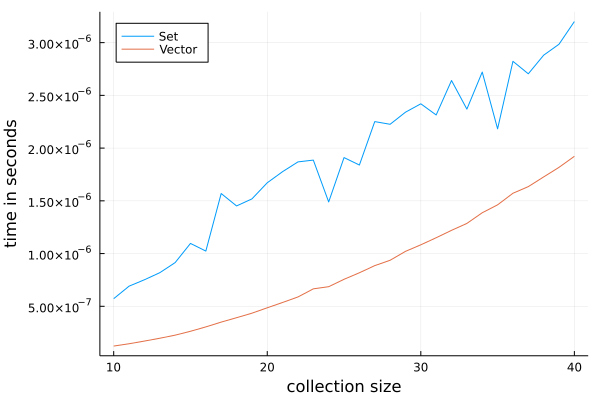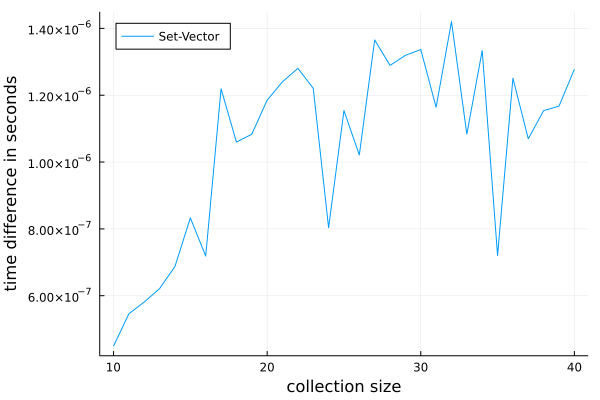# Introduction

Several years ago I switched to Julia because of its speed. However, every day I am positively surprised how fast it is.

Today I was writing a simulation where I needed to check if some value was contained in some collection. Traditionally programmers are recommended to use the Set structure to perform such operation. However, since I needed to squeeze out every last bit of performance in my code I investigated all available options. Julia developers positively surprised me as it seems that they managed to implement an extremely fast lookup in a standard Vector type.

Therefore in my post today I decided to share some benchmark results showing how fast lookup in Vector container is against Set lookup.

The post was written under Julia 1.7.0, DataFrames.jl 1.3.2, BenchmarkTools.jl 1.3.1, and Plots.jl 1.27.1.

# The experiment

In order to test scalability of lookup I decided to test the performance for collections from size 10 to 40 to see if some trend can be observed. I wanted to store in them random strings and then perform a check if every value stored in the collection is indeed present in it.

To ensure fair evaluation of the results I used the @belapsed macro from the BenchmarkTools.jl package. Here is the test code:

using DataFrames
using BenchmarkTools
using Random
using Plots

f(x, r) = all(in(x), r) # function testing lookup speed

df = DataFrame()
Random.seed!(1234)

for i in 10:40
v = [randstring(rand(1:1000)) for _ in 1:i] # randomly generate a Vector
s = Set(v) # turn it to Set for comparison
@assert length(s) == i # make sure we had no duplicates
t1 = @belapsed f($s,$v)
t2 = @belapsed f($v,$v)
# display the intermetiate results so that I can monitor the progress
@show (i, t1, t2)
push!(df, (;i, t1, t2))
end

plot(df.i, [df.t1 df.t2];
labels=["Set" "Vector"],
legend=:topleft,
xlabel="collection size",
ylabel="time in seconds")


The code produces the following plot:In the plot it seems that performance of function f doing lookup for both collections scales approximately linearly in the tested range. The lookup in Set is slower than the lookup in Vector. Additionally, which is positive, lookup time in Vector has a more stable timing (Set timings are strangely jagged). Finally, it seems that the difference in the timing increases with collection size. Let us visualize this:

plot(df.i, df.t1-df.t2;
labels="Set-Vector",
legend=:topleft,
xlabel="collection size",
ylabel="time difference in seconds")Indeed it seems that the timing difference increased in the tested range of collection sizes.

# Conclusions

It is getting late today, and I do not want to delay my weekly posts. Therefore, I will do some more testing in the coming week and report you the results in my next post.

From today’s experiments we can see that in the test setting I used Vector lookup was significantly faster than Set lookup.

As a side note, it was super easy to implement these benchmarks in Julia.